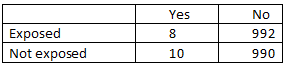## Chapter 128 Critical Appraisal of Literature Q&A (1 of 6) Sample Questions

1. From the above table what is risk reduction (RR)?
View Image Hide Image1. A. 80%
2. B. 0.8
3. C. 10%
4. D. 500
Ans: B
Tips: Control event rate (CER) = c/c + d
Experimental event rate (EER) = a/a + b
Relative Risk (RR) = EER/CER = (a/a+ b)/(c/c + d)
RR = (8/1000) / (10/1000) = 0.8